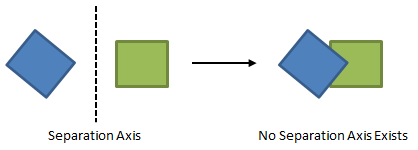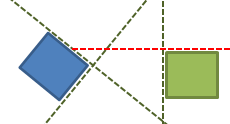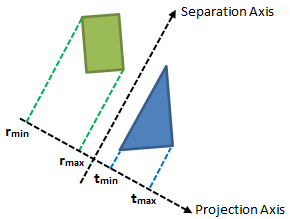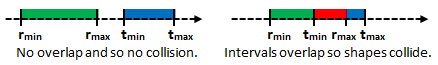## Friday, 4 May 2012

### 2D Collision Series : SAT Part 1

In this series, I will be giving a very brief insight into the world of 2D collision detection and response. Normally during collision detection there are two phases, Broad phase and Narrow phase.

This series will focus on the Narrow phase.

The Broad phase is where you quickly look over all of the objects in your world and do a very rough collision test (e.g Rectangle to Rectangle or Sphere to Sphere) to find pairs of objects that might be colliding. This step is performed first to help cut down on the number of more accurate, more expensive tests we will perform in the Narrow phase. The Broad phase is normally speeded up using spatial partitioning methods such as Quadtrees, however I will not be discussing such things in this series.

The Narrow phase is where we will look at each pair of possibly colliding objects found in the Broad Phase and do a more accurate check to test if the objects are actually colliding. In a lot of situations rectangles and spheres are not good enough to represent the shape of an object, what if we want to check if a rectangle
and a triangle are colliding for instance?. This will be the focus of this tutorial series.

In particular the method we will be using for our Narrow phase tests is called the Separating Axis Theorem (or SAT for short). Do not let the name put you off, the method itself is actually very simple to understand and only uses very little vector math!

Quite simply, SAT states that if we can draw a straight line between two convex shapes, the two shapes are not colliding. We refer to this line as the Separation Axis. Simple enough right?So which set of axes should we test and how do we actually test if an axis actually separates two shapes?

It would be naive to just keep picking lines at random and hope that one of them separates the two shapes; It turns out the only axes that we need to test are the ones that are parallel to the shapes edges, take the above rectangles for example:We would only need to test a total of 4 different axes to see if they separate the two shapes.

This answers the question of which axes do we look at, but now we need a way to test if an axis actually separates the two shapes.

To do this we ‘project’ each shape onto the axis which is perpendicular to the axis we are testing.
So what does this mean exactly? We first rotate our separation axis by 90° so we get an axis which is perpendicular to the original axis, we will call this rotated axis the Projection Axis.

Doing this with vectors is very simple, we simply multiply the axis Y component by –1 and then just swap the X and Y components:

We then project the shapes onto the projection axis; We can imagine this as us drawing a line from each vertex in each shape to the projection axis like in the following diagram:When we project a vertex onto the projection axis, we end up with a number which tells us how far along the axis the line we drew crosses. This number is called the dot (or sometimes the scalar) product of the vertex and the projection axis.

As you can see from the diagram above, the biggest and smallest numbers will give us an interval on the projection axis which will represent the projected shape (e.g. rmin and rmax define the interval of the rectangle).

By using these intervals we turn a daunting 2D problem into a nice and simple 1D problem:To check if the axis we are testing separates the two shapes, we simply need to check whether their intervals on the projection axis overlap! In pseudo code the checks would look something like this:

```if (tmin > rmax || rmin > tmax)
{
No collision!
}
else
{
Collision occured!
}```

And that’s all there is to SAT!

One of the big bonuses of using SAT is that it makes resolving a collision very easy for us! We already know how much the two shapes intersect each other by, so to make them stop intersecting we simply push each shape either forwards or backwards along the projection axis a distance equal to the amount of overlap / 2. (I will speak more on this in the next tutorial).

And that concludes part 1, in part two we will be turning the method explained above into actual useable code! I hope you have enjoyed this tutorial and have found it useful! If anything wasn’t clear or was explained badly please let me know and I'll try to elaborate.

1.So this works with any convex shape. Awesome
Looking forward to part two.

2.These days, there are different kinds of games for each age group and the progress in technology has provided a wide range of gadgets that offer endless entertainment to children. Children's games are particularly designed to provide fun and entertainment according to the requirements of each age group of children. Most games are created in order to help improve the creativity and skills of kids.
cheap elo boost
Cheap League of Legends Coaching

3.Such posts are very interesting. It keeps an interest of a person intact. I must forward this post with my best friend because her exam is around the corner and she will understand everything written here. I was just looking for some nice LSAT Logic Games online.

4.If you follow the instructions given then you can increase your elo and get some great games to play with. Once you get more games you can then begin to research them online to see what the sites do. Get more information about valorant boosting click here now.